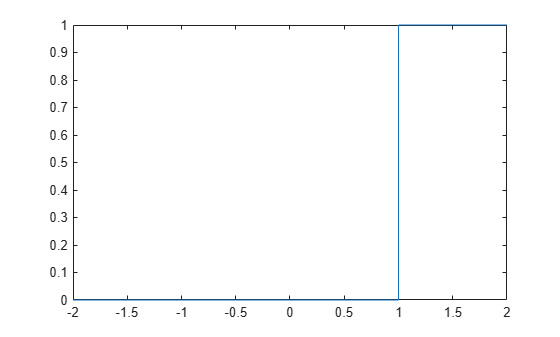# heaviside

Heaviside step function

## Syntax

``H = heaviside(x)``

## Description

example

````H = heaviside(x)` evaluates the Heaviside step function (also known as the unit step function) at `x`. The Heaviside function is a discontinuous function that returns `0` for `x < 0`, `1/2` for `x = 0`, and `1` for ```x > 0```.```

## Examples

collapse all

The `heaviside` function returns 0, 1/2, or 1 depending on the argument value. If the argument is a floating-point number (not a symbolic object), then `heaviside` returns floating-point results.

Evaluate the Heaviside step function for a symbolic input `sym(-3)`. The function `heaviside(x)` returns `0` for `x < 0`.

`H = heaviside(sym(-3))`
`H = $0$`

Evaluate the Heaviside step function for a symbolic input `sym(3)`. The function `heaviside(x)` returns `1` for `x > 0`.

`H = heaviside(sym(3))`
`H = $1$`

Evaluate the Heaviside step function for a symbolic input `sym(0)`. The function `heaviside(x)` returns `1/2` for `x = 0`.

`H = heaviside(sym(0))`
```H =  $\frac{1}{2}$```

For a numeric input `x = 0`, the function `heaviside(x)` returns floating-point results.

`H = heaviside(0)`
```H = 0.5000 ```

`heaviside` takes into account assumptions on variables.

Create a symbolic variable `x` and assume that it is less than 0.

```syms x assume(x < 0)```

Evaluate the Heaviside step function for the symbolic input `x`.

`H = heaviside(x)`
`H = $0$`

For further computations, clear the assumptions on `x` by recreating it using `syms`.

`syms x`

Plot the Heaviside step function for `x` and `x - 1`.

```syms x fplot(heaviside(x), [-2, 2])````fplot(heaviside(x - 1), [-2, 2])`Evaluate the Heaviside function for a symbolic matrix. When the input argument is a matrix, `heaviside` computes the Heaviside function for each element.

```syms x H = heaviside(sym([-1 0; 1/2 x]))```
```H =  $\left(\begin{array}{cc}0& \frac{1}{2}\\ 1& \mathrm{heaviside}\left(x\right)\end{array}\right)$```

Compute derivatives and integrals of expressions involving the Heaviside function.

Find the first derivative of the Heaviside function. The first derivative of the Heaviside function is the Dirac delta function.

```syms x diff_H = diff(heaviside(x),x)```
`diff_H = $\delta \text{dirac}\left(x\right)$`

Evaluate the integral ${\int }_{-\infty }^{\infty }{\mathit{e}}^{-\mathit{x}}\mathit{H}\left(\mathit{x}\right)\text{\hspace{0.17em}}\mathit{dx}$.

```syms x int_H = int(exp(-x)*heaviside(x),x,-Inf,Inf)```
`int_H = $1$`

Find the `fourier` transform of the Heaviside function.

```syms x F = fourier(heaviside(x))```
```F =  $\pi \delta \text{dirac}\left(w\right)-\frac{\mathrm{i}}{w}$```

Find the `laplace` transform of the Heaviside function.

```syms x L = laplace(heaviside(x))```
```L =  $\frac{1}{s}$```

The default value of the Heaviside function at the origin is `1/2`.

`H = heaviside(sym(0))`
```H =  $\frac{1}{2}$```

Other common values for the Heaviside function at the origin are 0 and 1. To change the value of `heaviside` at the origin, use `sympref` to set the value of the `'HeavisideAtOrigin'` preference. Store the previous parameter value returned by `sympref`, so that you can restore it later.

`oldparam = sympref('HeavisideAtOrigin',1);`

Check the new value of `heaviside` at `0`.

`H = heaviside(sym(0))`
`H = $1$`

The preferences set by `sympref` persist throughout your current and future MATLAB® sessions. To restore the previous value of `heaviside` at the origin, use the value stored in `oldparam`.

`sympref('HeavisideAtOrigin',oldparam);`

Alternatively, you can restore the default value of `'HeavisideAtOrigin'` by using the `'default'` setting.

`sympref('HeavisideAtOrigin','default');`

## Input Arguments

collapse all

Input, specified as a number, symbolic number, variable, expression, function, vector, or matrix.